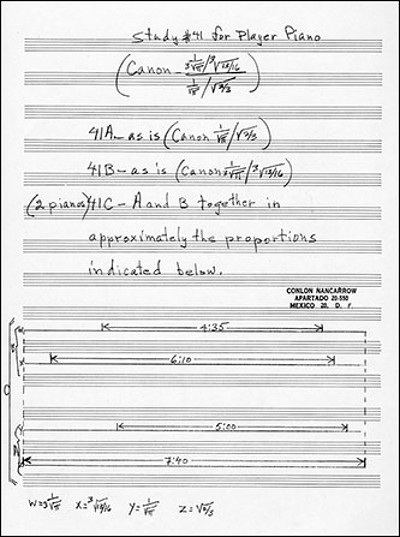# All Together NowBecause he wrote for player pianos, Conlon Nancarrow could demand more exacting performances than other composers. His Study No. 41 is in three parts for two pianos. The first canon has a time signature of$\frac{1}{\sqrt{\pi}} / \sqrt{2/3}$ for the first piano; the second is in$\frac{1}{3 \sqrt{\pi}} / \sqrt{13/16}$ for the second piano; and the third is in$\frac{\frac{1}{3 \sqrt{\pi}} / \sqrt{13/16}}{\frac{1}{\sqrt{\pi}} / \sqrt{2/3}}$ for both pianos. In the third part, the two performances must be played in proportions marked in a diagram in the score (above). It sounds like this: